# Summary of Equations of Motion for Rigid Bodies (Part - 1) Civil Engineering (CE) Notes | EduRev

## Civil Engineering (CE) : Summary of Equations of Motion for Rigid Bodies (Part - 1) Civil Engineering (CE) Notes | EduRev

The document Summary of Equations of Motion for Rigid Bodies (Part - 1) Civil Engineering (CE) Notes | EduRev is a part of the Civil Engineering (CE) Course Introduction to Dynamics and Vibrations- Notes, Videos, MCQs.
All you need of Civil Engineering (CE) at this link: Civil Engineering (CE)

In this section, we collect together all the important formulas from the preceding sections, and summarize the equations that we use to analyze motion of a rigid body.

We consider motion of a rigid body that has mass density ρ during some time interval t0 < t < t1 , and define the following quantities:

6.6.1 Forces, torques, impulse, work, power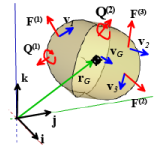• The total force acting on the body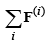• The total linear impulse exerted by forces during the time interval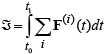• The total moment (including torques) acting on the body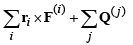• The tot al angular impulse exerted on the body during the time interval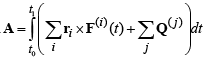• The rate of work done by forces and torques acting on the body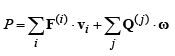• The total work done by forces and torques on the body during the time interval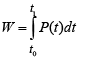6.6.2 Inertial properties

• The total mass is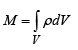• The position of the center of mass is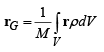•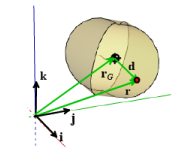• The mass moment of inertia about the center of mass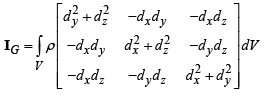where d = r − rG

For a 2D body with mass per unit area µ we use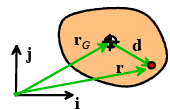• The total mass is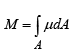• The position of the center of mass is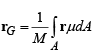• The mass moment of inertia about the center of mass is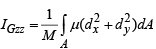where d = r − r

6.6.3 Describing motion

• The rotation tensor (matrix) maps the vector connecting two points in a solid before it moves to its position after motion rB − rA = R(pB − pA)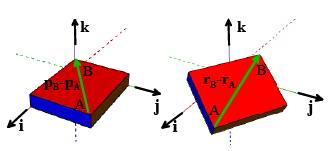• The spin tensor is related to R by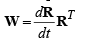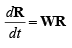• Rotation through an angle θ about an axis parallel to a unit vector  n = nx i +ny j + nzk is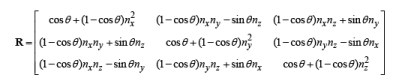• The angular velocity vector ω = ω x i +ωy j+ ω zk is related to W by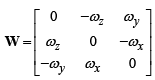• The angular acceleration vector is α = dω/dt
• The velocities of two points A and B in a rotating rigid body are related by v B −v A = ω ×(rB − rA )
• The accelerations of A and B are related by aB − aA = α ×(rB−rA) + ω×(vB − vA) = α ×(rB−rA) + ω× ω×(rB−rA)]

6.6.4 Momentum and Energy

• The total linear momentum is p = MvG
• The angular momentum (about the origin) is h =rG × MvG+ IG ω
• The total kinetic energy is T= 1/2 MvG ·vG +1/2 ω·IGω

For 2D planar problems, we know ω = ωzk .   In this case, we can use

• The total linear momentum is p = MvG
• The total angular momentum (about the origin) is h =rG × MvG+IGzzω z k
• The total kinetic energy is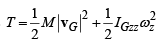6.6.5 Conservation laws

• Linear momentum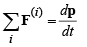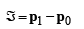• Angular momentum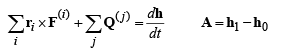• Work-Power - Kinetic Energy relation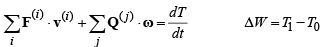• Energy equation for a conservative system d/dt (T+V) =0  T0+ V0= T1+V1

Offer running on EduRev: Apply code STAYHOME200 to get INR 200 off on our premium plan EduRev Infinity!

## Introduction to Dynamics and Vibrations- Notes, Videos, MCQs

20 videos|53 docs

,

,

,

,

,

,

,

,

,

,

,

,

,

,

,

,

,

,

,

,

,

;